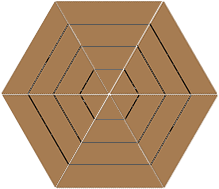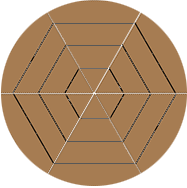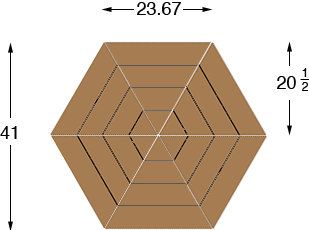SEARCH HOMEMath Central Quandaries & QueriesQuestion from Pat: I need to rebuild a wagon wheel, the metal wheel rim is 41" diameter, inside the rim is a one and a half inch by one and a half inch wood wheel. I thought I would glue up a hexagon from a 2x6 or 2x8 piece of wood and then draw and cut out the 41" diameter wood circle. ? what would work better the 2x6 or the 2x8 and what is the length of the cuts needed in order to give me the 41' diameter I need.Hi Pat,

Is this what you have in mind to build,and then cut out the circle?If my diagrams are correct then using the analysis in Steve's answer to an earlier question the length of each side of the hexagon is 23.67 inches.I have each triangle made from 4 boards and 20 1/2 divided by 4 is 5 1/8 so this can be ripped from a 2 by 6. But 20 1/2 divided by 3 is about 6 7/8 so if you use 2 by 8s the triangles can be made from 3 boards.

I hope this helps,
HarleyMath Central is supported by the University of Regina and The Pacific Institute for the Mathematical Sciences.